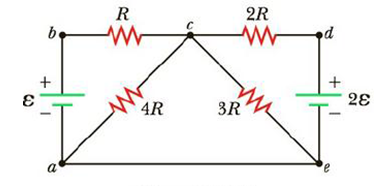Chapter 18, Problem 21P

Chapter
Section
Textbook Problem

Taking R = 1.00 kΩ and ε = 250 V in Figure P18.21, determine the direction and magnitude of the current in the horizontal wire between a and e.Figure P18.21

To determine
The magnitude of the current and the direction in the horizontal wire between a and e.

Explanation

Given Info: Voltage ε is 250V and resistance R is 1.00 .

Explanation:

The below figure shows the direction of current flow in the circuit.

From the above figure,

• ε is voltage in the circuits
• R is corresponding resistance in the circuits
• I,I1,I2 is corresponding current in the circuits

Consider, the current passing from c to a is I1 , the current passing from c to e is I2 ,

the current passing from a to e is I voltage is ε and resistance is R

Apply Kirchhoff’s voltage law to the left and right loop.

From the left loop abca,

εR(I1I)4RI1=0ε+RI=RI1+4RI1I1=ε+RI5R=15(εR+I)

From the right loop cedc,

3RI2+2ε2R(I2+I)=05RI2=2ε2RII2=2(εRI)5R=25(εRI)

Conclusion:

Formula to calculate current by using Kirchhoff’s junction rule, to caec

4RI1+3RI2=04RI1=3RI2

Use 1/5[(ε/R)+I] for I1 and (2/5)[(ε/R)I] for I2 in the above equation to find expression for I

Still sussing out bartleby?

Check out a sample textbook solution.

See a sample solution

The Solution to Your Study Problems

Bartleby provides explanations to thousands of textbook problems written by our experts, many with advanced degrees!

Get Started